GCSE Maths Geometry and Measure

Loci And Construction

# Loci And Construction

Here we will learn about loci, constructions and bearings, including drawing various constructions, using constructions when drawing loci and measuring and calculating bearings.

There are also loci & construction worksheets based on Edexcel, AQA and OCR exam questions, along with further guidance on where to go next if you’re still stuck.

## What are loci and construction?

Loci and construction are geometric objects that are drawn with specific features.

To draw loci and constructions accurately, you will be required to use a ruler, a pair of compasses, and a protractor when appropriate.

• A ruler is used to measure distances and construct straight lines.
• A pair of compasses is used to draw points that are a fixed distance from a point.
• A protractor is used to measure and construct angles.

Constructions are set methods for creating accurate drawings in maths.

A locus of points (plural loci) is a set of points that follow a given rule. We often use constructions to accurately draw loci.

A bearing is an angle, measured clockwise from north, used to describe the position of an object. Bearings are given using three figures, for example 052^{\circ}.

### What are loci and construction?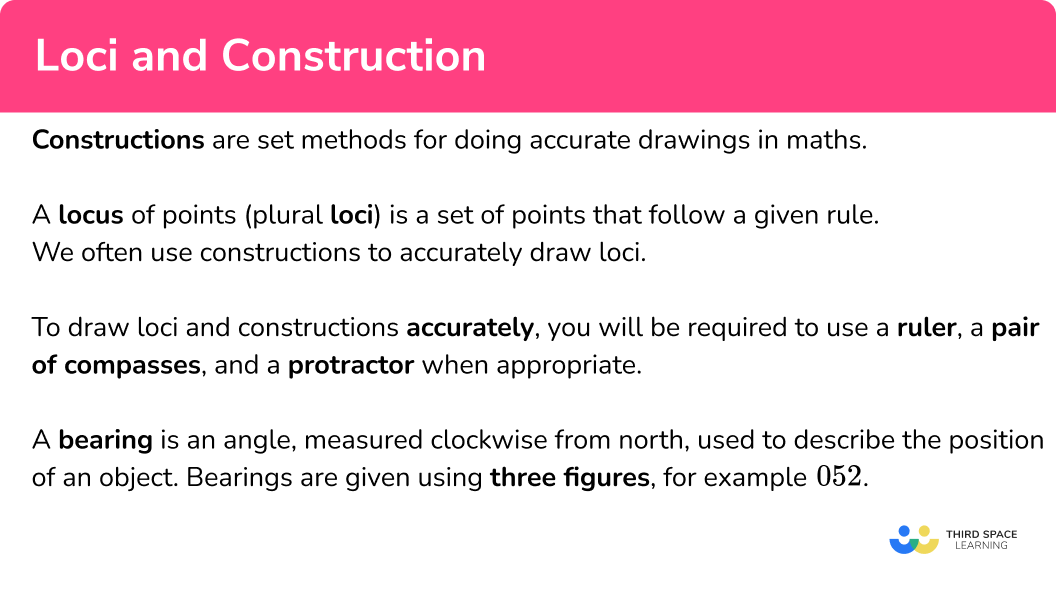### ● Constructions

There are a number of different constructions that you need to be able to perform for GCSE maths. These include,

• Angle bisector
• Perpendicular bisector of a straight line
• Perpendicular line to or from a particular point
• Triangles, given information about sides and/or angles
• Polygons

To do constructions we carefully follow the method, leaving all construction lines visible.

The most common constructions are,

When doing constructions, all construction lines should be left visible.

Step-by-step guide: Constructions

### ● Loci

A locus of points (plural loci) is a set of points that follow a given rule. Given a rule, we need to be able to identify the points that follow that rule.

Here are some examples,

Step-by-step guide: Loci

### Bearings

A bearing is an angle, measured clockwise from north, used to describe the position of an object. They are written using three figures. Bearings can be drawn, measured or calculated depending on the problem.

The bearing of B from A is 075^{\circ}. This means to get from A to B, you need to travel in a direction 75^{\circ} clockwise from north.

We can find the bearing of A from B using angle rules.

As both north lines in the diagram below are in the same direction, these lines are parallel. This means that the angle at A and the angle at B are co-interior (their angle sum is 180^{\circ} ).

The angle x is equal to 180-75=105^{\circ}.

The back bearing is the bearing of A from B. As the sum of angles at a point is 360^{\circ}, the bearing \theta of A from B in the diagram below is 360-105=255^{\circ}.

Step-by-step guide: Bearings

## How to use loci and constructions

### How to use loci and constructions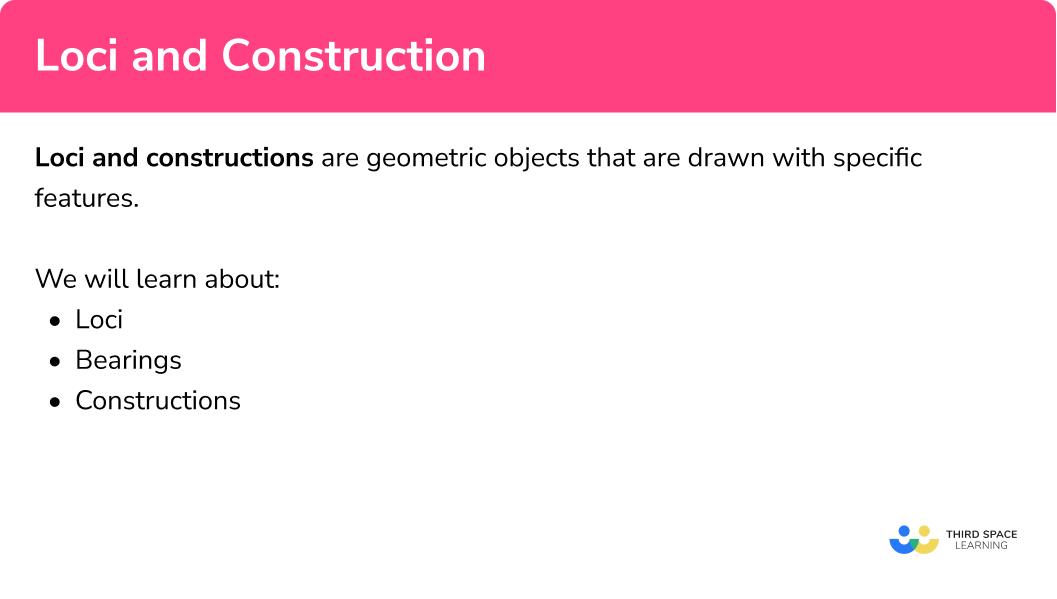## Loci and construction examples

### Example 1: bisect an angle

Bisect the angle ABC.

1. With the point of the compasses on the vertex, draw an arc.

2With the point of the compasses on each intersection, draw two further arcs.

3Draw a line from the vertex to the intersection of the arcs.

Step-by-step guide: Angle bisector

### Example 2: locus of points equal distance from two points (perpendicular bisector)

Draw the locus of points equidistant from lighthouse A and lighthouse B

The locus of points equidistant from two points is the perpendicular bisector of the line between those points. Imagine a straight line connecting the two points A and B.

We need to construct the perpendicular bisector of the line AB

With the point of the compasses on one end of the line, draw an arc.

With the point of the compasses on the other end of the line, draw a second arc.

Draw a straight line through the two points where the arcs cross.

Step-by-step guide: Perpendicular bisector

### Example 3: combined loci problem (distance from a point)

Two phone masts, R and S are 50 \ km apart. The signal from phone mast R can reach 30 \ km and the signal from phone mast S can reach 35 \ km. Using the scale 1 \ cm=10 \ km, shade the area covered by signal from both phone masts.

Use the wording of the region required to decide what constructions are needed.

Perform any relevant constructions for points or line segments involved.

Indicate the region required as necessary.

Step-by-step guide: Constructions between points and lines

### Example 4: combined loci problem (distance from a line)

Below is a diagram of Ellie’s garden. Ellie wants to plant a new tree. She wants the tree to be closer to the side AD than the side AB, more than 2 \ m from A and less than 3 \ m from the side AB. Using the scale 1 \ cm=1 \ m, indicate the area in which Ellie can plant the tree.

Use the wording of the region required to decide what constructions are needed.

Perform any relevant constructions for points or line segments involved.

Indicate the region required as necessary.

### Example 5: draw a bearing

The bearing of a ship from lighthouse A is 130^{\circ}. The bearing of the same ship from lighthouse B is 025^{\circ}. Mark the position of the ship on the diagram.

Locate the point you are measuring the bearing from and draw a north line if there is not already one given.

Using your protractor, place the zero of the scale on the north line and measure the required angle clockwise, make a mark on your page at the angle needed.

Draw a line from the start point in the direction of the bearing. If you are producing a scale drawing and know the distance to locate a point use this scale appropriately.

### Example 6: calculate a bearing

A plane starts at airport A. The plane flies 40 \ km east and then 60 \ km north before landing at airport B. Find the bearing of airport B from airport A.

Locate the points you are calculating the bearing from and to.

Using the north lines for reference at both points, use angle rules and/or trigonometry to calculate any angles that are required.

Read off the three-figure bearing required.

Step-by-step guide: Constructing triangles

### Common misconceptions

• Removing construction lines

When drawing constructions, the construction lines must be shown and not erased.

• Corners when drawing the locus around a polygon or line

When drawing the locus around a polygon or a line, the locus around a vertex should be an arc. For example,

• Connecting the corners instead of bisecting an angle

When finding the locus of points equidistant from two sides of a rectangle, we need to bisect the angle rather than drawing a line between the two corners.

For example,

• Not measuring bearings from north

It is important to remember that bearings are always measured from north.

• Not measuring bearings in a clockwise direction

Bearings must be measured in a clockwise direction.

For example, the bearing of A from B is 360-100=260^{\circ}, not 100^{\circ}.

### Practice loci and construction questions

1. What type of construction is shown below?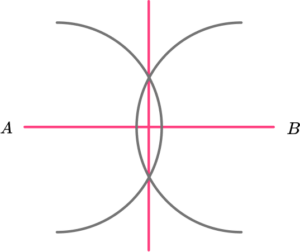Angle bisector of the angle ABPerpendicular to the line AB at the point PPerpendicular bisector of the line ABTriangle ABCThe two straight lines are at right angles to each other and so they are perpendicular lines. Also, the radius of each arc from A and B is the same and so the line is bisected into two equal halves.

2. Which diagram correctly shows how to construct the angle bisector of angle ABC?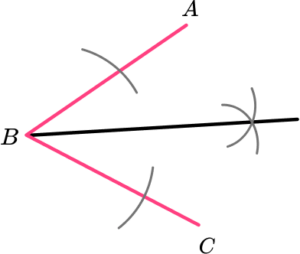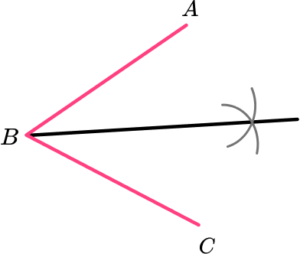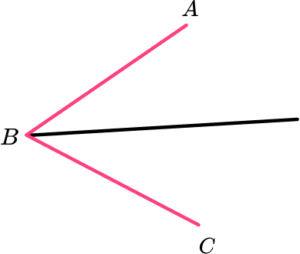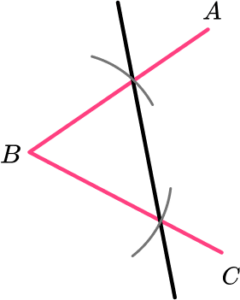An arc of the same radius is drawn on line AB and BC from the point B. From these arcs, two intersecting arcs are drawn with a straight line connecting the vertex at B and the intersection point.

3. Which diagram shows the locus of points a distance of d from the triangle?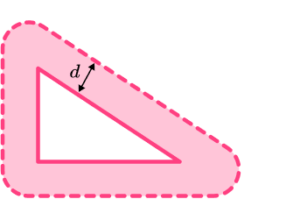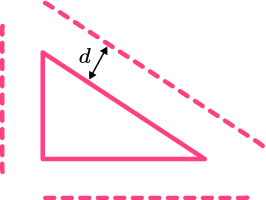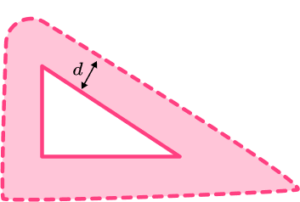Each vertex should be treated as a point and therefore the locus around each vertex should be an arc. The locus of points from a line are parallel.

4. Hannah wants to shade the area closer to side AB than side AD, and more than 2 \ cm from point B. Which diagram shows the correct area?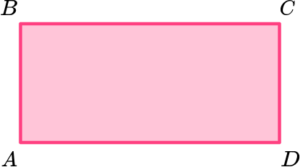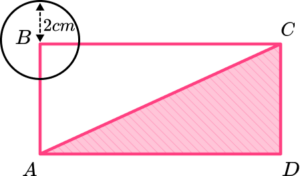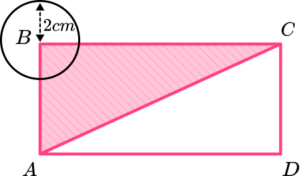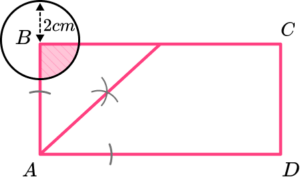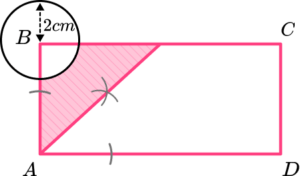We need to draw a circle with radius 2 \ cm around the point B and the angle bisector of angle BAD. The shaded region is outside of the circle and to the left side of the line drawn for the angle bisector.

5. Find the bearing of P from Q.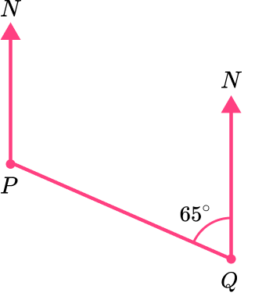065^{\circ}115^{\circ}295^{\circ}025^{\circ}Bearings are measured clockwise from north. The bearing of P from Q is 360-65=295^{\circ}.

6. The bearing of airport B from airport A is 100^{\circ}. What is the bearing of airport A from airport B?

260^{\circ}280^{\circ}80^{\circ}190^{\circ}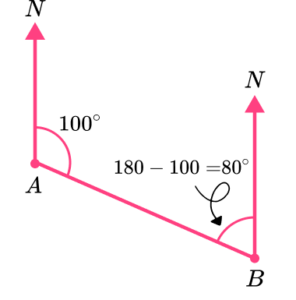By drawing a diagram, we can see that the co-interior angle at B is 180-100=80^{\circ} (remember that all north lines are parallel). Therefore the bearing of A from B, measured clockwise from north, is 360-80=280^{\circ}.

### Loci and construction GCSE questions

1. Construct the perpendicular to the line AB at the point P.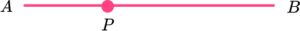(3 marks)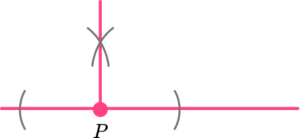Two arcs equidistant from P.

(1)

Two further arcs above (or below) P.

(1)

Perpendicular drawn and all construction lines shown.

(1)

2. Shade the area that is closer to the side AB than the side BC and is closer to the point B than the point A.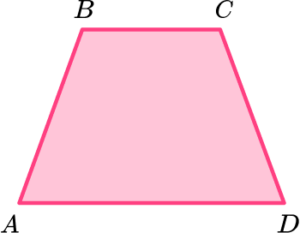(3 marks)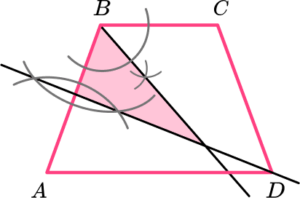Perpendicular bisector of AB.

(1)

Angle bisector of angle ABC.

(1)

(1)

3. Some rangers are tracking the position of a lion.

The lion is on a bearing of 055^{\circ} from ranger A and 290^{\circ} from ranger B.

Mark the position of the lion on the diagram below.(3 marks)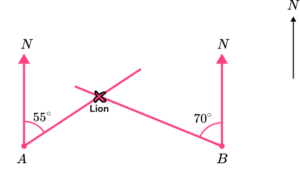Bearing of 055^{\circ} from A drawn.

(1)

Bearing of 290^{\circ} from B drawn.

(1)

Position of lion marked.

(1)

## Learning checklist

You have now learned how to:

• Derive and use the standard ruler and compass constructions (perpendicular bisector of a line segment, constructing a perpendicular to a given line from/at a given point, bisecting a given angle); recognise and use the perpendicular distance from a point to a line as the shortest distance to the line
• Interpret and use bearings

## Still stuck?

Prepare your KS4 students for maths GCSEs success with Third Space Learning. Weekly online one to one GCSE maths revision lessons delivered by expert maths tutors.

Find out more about our GCSE maths tuition programme.